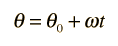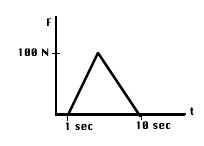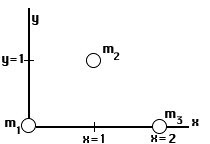## Wednesday, January 23, 2008

### Torque

imagesTorque is a measure of how much a force acting on an object causes that object to rotate. The object rotates about an axis, which we will call the pivot point, and will label 'O'. We will call the force 'F'. The distance from the pivot point to the point where the force acts is called the moment arm, and is denoted by 'r'. Note that this distance, 'r', is also a vector, and points from the axis of rotation to the point where the force acts.

Torque = r x F = r F sin(theta)
There may be more than one force acting on an object, and each of these forces may act on different point on the object. Then, each force will cause a torque. The net torque is the sum of the individual torques.
Rotational Equilibrium is analogous to translational equilibrium, where the sum of the forces are equal to zero. In rotational equilibrium, the sum of the torques is equal to zero. In other words, there is no net torque on the object.
The SI units of torque is a Newton-metre.

### Angular Velocity

imageAll points in a rigid object rotate with the same angular velocity.
An object such as a wheel rotates about a fixed axis either clockwise or counterclockwise.
The direction can be specified with a + or - sign.

## Monday, October 8, 2007

### Angular Quantites

http://hyperphysics.phy-astr.gsu.edu/hbase/circ.htmlIn purely rotational motion, all points on the object move in circles around the axis of rotation (“O”). The radius of the circle is r. All points on a straight line drawn through the axis move through the same angle in the same time. The angle θ in radians is defined:

s = r θ

where s is the arc length.

### Rotational Motion (Chapter 8)

Objectives

1. Convert angular quantities from revolutions or degrees to radians and vice versa.

2. Write the Greek symbols used to represent angular displacement, angular velocity, and angular acceleration.

3. State the meaning of the symbols used in the kinematics equations for uniformly accelerated angular motion.

4. Write from memory the equations used to describe uniformly accelerated angular motion.

5. Complete a data table using information both given and implied in word problems. Use the completed data table to solve word problems related to angular kinematics.

6. Distinguish between inertia and moment of inertia.

7. Distinguish between linear momentum and angular momentum. State and apply the law of conservation of angular momentum to solve word problems.

8. Calculate the lever arm distance and determine the magnitude and direction of the torque vector if the magnitude and direction of the net force are given.

9. Draw a free body diagram for each object in a system. Locate the forces acting on each object. Use F = ma and τ = Iα to solve for the linear or angular acceleration of each object.

10. Apply the law of conservation of angular momentum to a system where no net external torque acts. Determine the change in angular velocity of a system where the moment of inertia of the objects that make up the system changes.

11. Distinguish between translational kinetic energy and rotational kinetic energy. Apply the Law of Conservation of Energy to solve problems that involve rotational as well as translational kinetic energy.

## Wednesday, October 3, 2007

### Practice Questions1. An Olympic diver dives off the high-diving platform. The magnitude of his momentum will be a maximum at Point

(A)A
(B)B
(C)C
(D) D

2. Which of the following can best be described as an elastic collision?
(A) A falling tree hitting the earth.
(B) A linebacker stopping a fullback.
(C) A golf club striking a golf ball.
(D) A pie in the face.

3. The Center of Mass of which of the following objects would not lie within the body itself?
(A) baseball
(B) brick
(C) frisbee
(D) paperback book4. What is the magnitude of the impulse as shown in the following figure?

(A)100 Ns
(B)900 Ns
(C)1000 Ns
(D)1100 Ns5. If the impulse is applied to a particle at rest, what will be its final momentum?

(A)100 kg m/s
(B)900 kg m/s
(C)1000 kg m/s
(D)1100 kg m/s6. What is the impulse shown in the following figure?

(A)225 Ns
(B)450 Ns
(C)900 Ns
(D) 1000 Ns7. If the impulse is applied to a 200 gm model rocket at rest, what will be its final speed?
(A) 2.25 m/s
(B) 22.5 m/s
(C) 2250 m/s
(D) 4500 m/s

8. What constant force acting over the same time as the force in Question 6 would produce the same impulse?
(A)45 N
(B) 50 N
(C) 90 N
(D)100 N

9. If each mass in the figure is 1 kg, what is the x location of the center of mass?

(A) .5
(B) .67
(C)1.0
(D) 1.3310. If each mass in the figure is 1 kg, what is the y location of the center of mass?
(A) .33
(B) .5
(C) .67
(D) .8511. If the mass of m1 and m3 in the figure is 1 kg and m2 is 2 kg, what is the x location of the center of mass?
(A) 1.33
(B) 1.0
(C) .67
(D) .3312. If the mass of m1 and m3 in the figure is 1 kg and m2 is 2 kg, what is the y location of the center of mass?
(A) .75
(B) .5
(C) .33
(D) .2513. If the mass of m1 is 1 kg and m3 is 2 kg in the figure and m2 is 3 kg, what is the x location of the center of mass?
(A) .67
(B) 1.17
(C) 1.33
(D) 1.6714. If the mass of m1 is 1 kg and m3 is 2 kg in the figure and m2 is 3 kg, what is the y location of the center of mass?
(A).25
(B).5
(C) .67
(D) .75

15. If all the masses in the figure have a mass of 2 kg, and m2 has speed 2 m/s and m3 has a speed of 4 m/s, what is the x-component of the momentum of the center of mass?

(A)2 kg m/s
(B)4 kg m/s
(C)6 kg m/s
(D)10 kg m/s16. If all the masses in the figure have a mass of 2 kg, and m2 has speed 2 m/s and m3 has a speed of 4 m/s, what is the y-component of the momentum of the center of mass?
(A)2 kg m/s
(B) 4 kg m/s
(C) 8 kg m/s
(D) 10 kg m/s17. What is the magnitude of the momentum of the center of mass of the system of particles in Question 15?

(A) 4kg m/s
(B) 8 kg m/s
(C) 8.9 kg m/s
(D)10 kg m/s

18. What is the direction of the momentum of the center of mass of the particles in Question 15, as measured in degrees counter clockwise from East?

(A) 15
(B) 34
(C) 45
(D) 63

19. A 12000 kg railroad car travelling at 10 m/s strikes and couples with a 6000 kg caboose at rest. What is the speed of the final combination?

(A) 3.3 m/s
(B)5.0 m/s
(C)6.7 m/s
(D)10 m/s

20. A 12000 kg railroad car travelling at 10 m/s strikes and couples with a 6000 kg caboose at rest. What is the loss of mechanical energy in the collision?

(A) 50000 J
(B) 100000 J
(C) 200000 J
(D) 250000 J

21. A 12000 kg railroad car travelling at 10 m/s strikes and couples with a 6000 kg caboose at rest. What would be the speed of the combination of railroad cars if the caboose was initially rolling towards the other car with a speed of 2 m/s?

(A) 0 m/s
(B) 6.0 m/s
(C) 7.3 m/s
(C) 12 m/s

22. A billiard ball travelling to the right at 6m/s overtakes and strikes an identical ball travelling at 3 m/s. What is the speed of the first ball after the collision?

(A) -6 m/s
(B) -3 m/s
(C) 3 m/s
(D) 6 m/s

23. A billiard ball travelling to the right at 6m/s overtakes and strikes an identical ball travelling at 3 m/s. What is the speed of the second ball after the collision?

(A)-6 m/s
(B) -3 m/s
(C) 3 m/s
(D) 6 m/s

24. A billiard ball travelling to the right at 6m/s overtakes and strikes an identical ball travelling at 3 m/s. What is the speed of the first ball after collision if the second ball was travelling at it (instead of away from it) with a speed of 3 m/s before collision?

(A) -6 m/s
(B) -3 m/s
(C) 3 m/s
(D) 6 m/s

25. What is the speed of the second ball in Question 24 after collision?

(A) -6 m/s
(B) -3 m/s
(C) 3 m/s
(D) 6 m/s